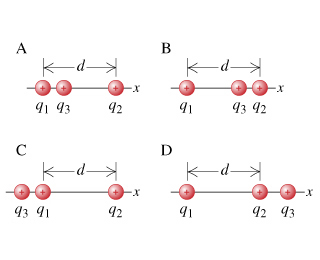# Problem: Two charged particles, with charges q1 = q and q2 = 4q, are located at a distance d = 2.00cm apart on the x-axis. A third charged particle, with charge q3 = q, is placed on the x-axis such that the magnitude of the force that charge 1 exerts on charge 3 is equal to the force that charge 2 exerts on charge 3. Find the position of charge 3 when q = 2.00 nC.Which of the following sketches represents a possible configuration for this problem?

###### FREE Expert Solution

In this problem, we are required to apply coulombs law and solve for the position of charges which gives zero net force on charge 3.

Coulomb's law:

95% (391 ratings)###### Problem Details

Two charged particles, with charges q1 = q and q2 = 4q, are located at a distance d = 2.00cm apart on the x-axis. A third charged particle, with charge q3 = q, is placed on the x-axis such that the magnitude of the force that charge 1 exerts on charge 3 is equal to the force that charge 2 exerts on charge 3. Find the position of charge 3 when q = 2.00 nC.

Which of the following sketches represents a possible configuration for this problem?